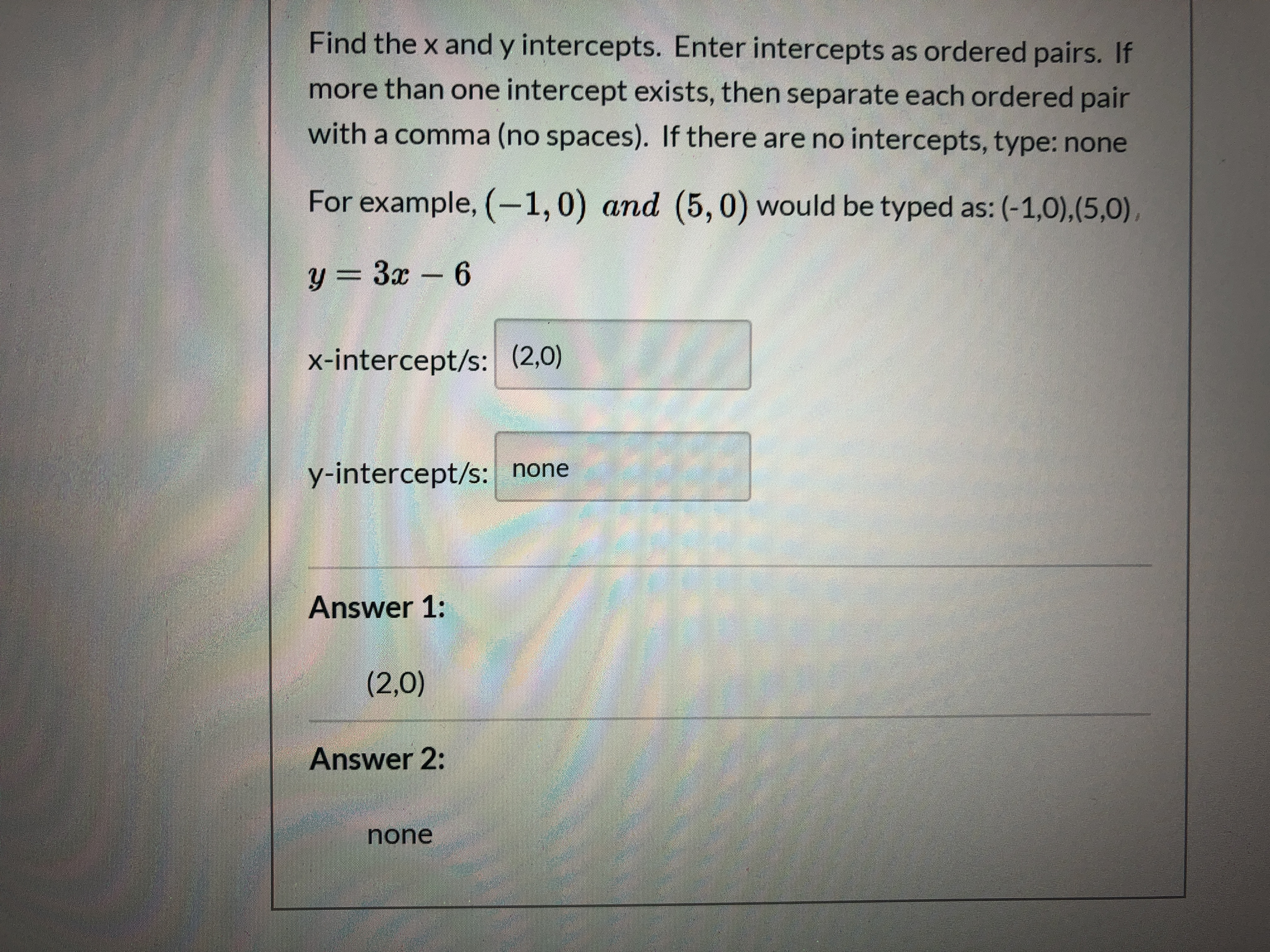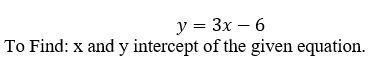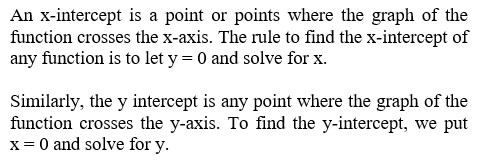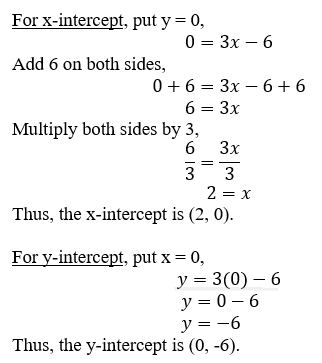# Find the x and y intercepts. Enter intercepts as ordered pairs. Ifmore than one intercept exists, then separate each ordered pairwith a comma (no spaces). If there are no intercepts, type: noneFor example, (-1,0) and (5, 0) would be typed as: (-1,0),(5,0),y = 3x - 6%3Dx-intercept/s: (2,0)y-intercept/s: noneAnswer 1:(2,0)Answer 2:none

Question
1 views

I keep getting wrong and I don't know whyhelp_outlineImage TranscriptioncloseFind the x and y intercepts. Enter intercepts as ordered pairs. If more than one intercept exists, then separate each ordered pair with a comma (no spaces). If there are no intercepts, type: none For example, (-1,0) and (5, 0) would be typed as: (-1,0),(5,0), y = 3x - 6 %3D x-intercept/s: (2,0) y-intercept/s: none Answer 1: (2,0) Answer 2: none fullscreen
check_circle

Given an equationNote:Now, using the definition we solve,...

### Want to see the full answer?

See Solution

#### Want to see this answer and more?

Solutions are written by subject experts who are available 24/7. Questions are typically answered within 1 hour.*

See Solution
*Response times may vary by subject and question.
Tagged in

### Algebra